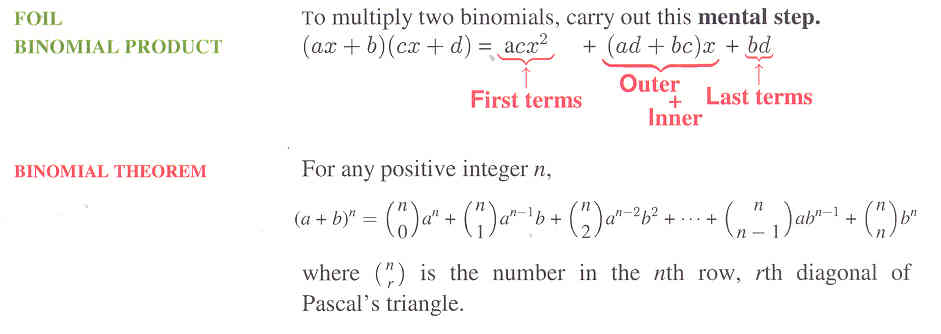# Section 6.1: Polynomials

## 6.1 Outline

1. Terminology
1. term
2. polynomial
1. monomial
2. binomial
3. trinomial
3. degree
1. of a term
2. of a polynomial
3. linear
4. numerical coefficient
2. Simplification
1. like terms/similar terms
2. simplify – this is the first of four main processes in algebra
3. Shortcuts with products
1. FOIL (see below)
2. binomial product
3. expand a binomial
4. Pascal’s triangle
4. Binomial theorem
5. Polynomials and areas
1. area of a square
2. area of a rectangle

Here is a graphic which explains the FOIL shortcut:## 6.1 Essential Ideas

The terminology associated with polynomials is important because it is used throughout all of
mathematics. Review these terms from this section: term, polynomial, monomial, binomial, trinomial, degree of a term, degree of a polynomial, linear, quadratic, numerical coefficient, like terms, and similar terms. Here are some additional essential ideas:

To simplify a polynomial means to carry out all operations (according to the order of operations agreement) and to write the answer in a form with the highest degree term first, with the rest of the terms arranged by decreasing degree. If there are two terms of the same degree, arrange those terms alphabetically.

Binomials are important in algebra. Here are two important
binomial ideas: